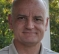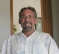## Setting required FFT spectrum bandwidth w.r.t the Sampling rateStarted by 2 years ago4 replieslatest reply 2 years ago328 views

I am working on an accelerometer with sampling rate of 26.7 kHz on which I intend to calculate FFT. Hence the FMax =  (26.7/2.56 = 10.4kHz). As I am performing this on a microcontroller, I have a limitation that I can only compute 2048-point FFT where my LOR(Lines of Resolution) is 2048/2.56 = 800.

Now the problem is that my deltaF = 10400/800 = 13Hz, which is a very low resolution hence I cannot go ahead with this solution.  Also my requirement is to calculate FFT upto 1 kHz where my deltaF will be 1000/800 = 1.25Hz, which is acceptable to me.

So is it possible to reduce FMax of my spectrum from 10.4 kHz to 1 kHz with the abovementioned sampling rate of the sensor?

FYI,

The sensor has an inbuilt LPF which I can implement at 1 kHz.

#FFT

[ - ]If you only care about signals up to 1kHz, you can filter and decimate the sample data down to a lower rate, and then run 2048 point FFTs on the lower sampling rate output.
If you decimate by 4, then your resulting data will be sampled at 10.4k/4 = 2.6 kHz.  A 2048 point FFT will give you bin resolution of 1.26953125 Hz.

Hope this is a useful idea.

If your input data are already filtered by a 1 kHz LPF, then you could just throw away 3 out of every 4 samples, and then do the 2048 point FFT.

[ - ]Why do you want to compute an FFT? Do you really need the entire spectrum?

If you only need the spectrum in a known area you can perform a zoom-FFT which has much higher resolution there.

If you only want to find the most significant component frequencies, then FFT is a really bad idea. Will a max entropy or Pisarenko (PHD) work for you? For a small number of lines they are efficient and give much higher resolution (dependent on SNR not on bin size).

[ - ]Hi,  you can try ZoomFFT or CZT (chirp z-transform) which can do DFT from f1 to f2 with higher resolution. Hope it helps.

Regards,

Chalil

[ - ]